WEBINAR
Brushless DC Permanent Magnet Motors: Design, Simulation, and Performance Evaluation
Thursday, October 19, 2023
Time
SESSION 1
SESSION 2
CEST (GMT +2)
03:00 PM
08:00 PM
EDT (GMT -4)
09:00 AM
02:00 PM
HOME / Applications / 2D FEM simulation of planar and axisymmetric DC actuators using EMWorks2D inside SOLIDWORKS

# 2D FEM simulation of planar and axisymmetric DC actuators using EMWorks2D inside SOLIDWORKS

Used Tools:## DC Actuators

Electromagnetic actuators are electromechanical components used to convert electrical power to a mechanical motion. they cover translational and rotational motion. DC actuators are generally composed of permanent magnets, solenoids and ferromagnetic parts. Solenoid actuators (Figure 1) have a steel armature which can move only in linear direction. The working principle consists of passing a DC current through a multi turn coil which can generate a static magnetic flux. The magnetic flux density will produce a magnetic force on the moving part (or the plunger). The plunger and the coil housing are made of high permeability ferromagnetic material to easily conduct the magnetic field.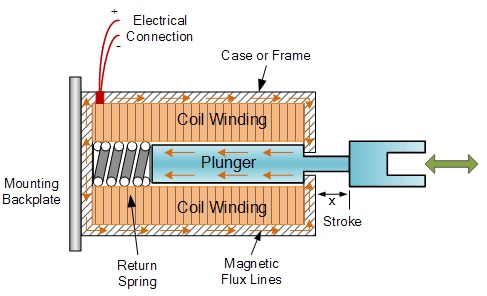Figure 1 - DC actuator .

FEM simulation is used to study and predict the magnetic fields and the force produced by the DC actuator at each plunger position.

## Two-dimensional approximation

Finite element method (FEM) has two variants: two dimensional (2D) and three dimensional (3D). A significant advantage of the 3D FEM is the capability of modeling full geometry of the analyzed object, including end-region of the windings. The 2D FEM allows only to calculate field distribution and other parameters in a cross-section of the machine model. Moreover, 2D simulation helps to predict results faster and offers more design iterations. On the other hand, 3D FEM offers more accurate and realistic results. However, computation time of the 3D model is much longer than the 2D one.

## Nonlinear magnetostatic simulation using EMWorks2D

### Example 1: Planar DC actuator 

A translational symmetric model  is proposed and analyzed using EMWors2D. Figure 2 shows the simulated 2D model. It is composed of a stator and a moving plunger; both are made of Silicon steel RM50 characterized by the BH curve shown in Figure 3, and a coil made of copper (1575 A-t).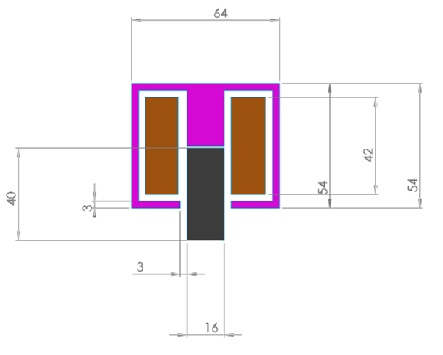Figure 2 - simulated model.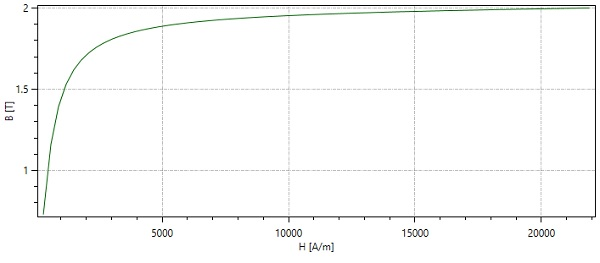Figure 3 - BH curve of Silicon steel RM50 .

Figure 4 shows the mesh of the model. The model is meshed with triangle elements. A smaller elementsize near the air gap are generated by adding a mesh control on the edges. Figure 5 and 6 show respectively fringe plot of the magnetic flux and the flux lines of the magnetic vector potential.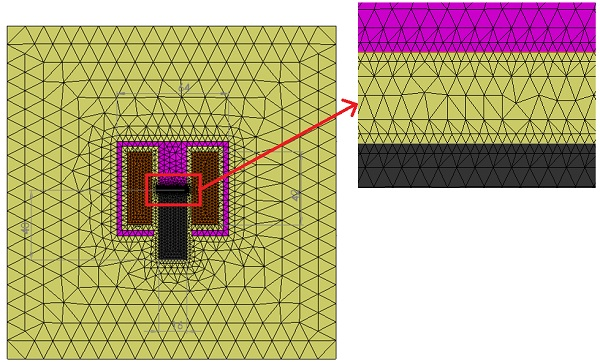Figure 4 - Meshed model.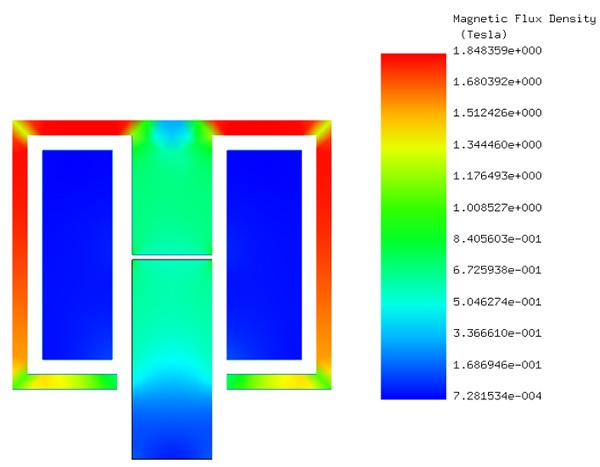Figure 5 - Fringe plot of the magnetic flux density.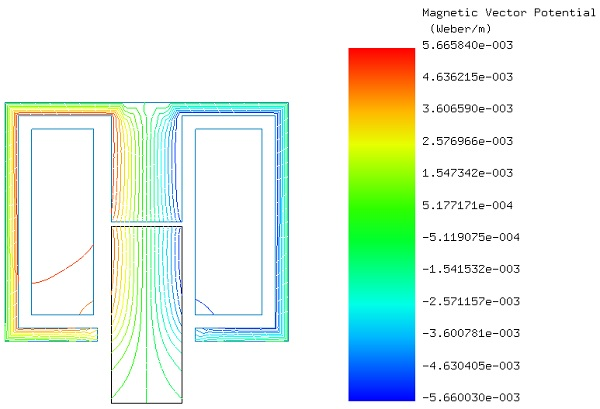Figure 6 - Flux lines of the magnetic vector potential.

The force generated by the actuator is computed versus air gap (Distance between plunger and stator). For this purpose, a parametric analysis is performed with EMWorks2D. It allows to parameterize both geometrical and simulation variables.

Figure 7 contains a comparison of the computed force given by EMWorks2D and ref . it shows a good agreement between both results. The force is inversely proportional to the air gap distance. i.e.the smaller the air gap distance is, the higher the magnetic force gets. This phenomenon can be simply explained by the behavior of the magnetic reluctance (magnetic resistance) which is proportional to the air gap distance.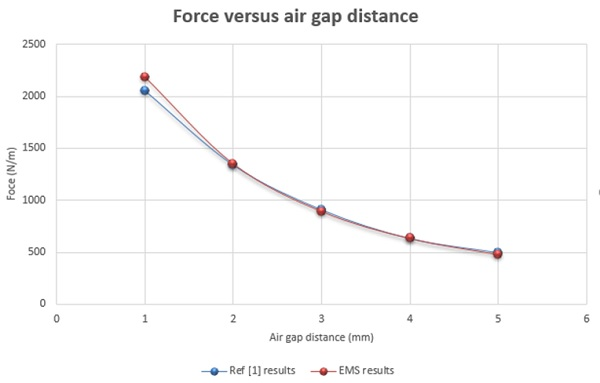Figure 7 - Force versus air gap distance.

### Example 2: Axisymmetric solenoid actuator 

A linear DC actuator with rotational axisymmetry is presented in Ref. In this example, the simulation is performed for the following two cases:

• The Stator and the moving plunger are made of nonlinear Carbon steel characterized by the BH curve shown in Figure 8.
• The gap between moving and non-moving components is filled with air.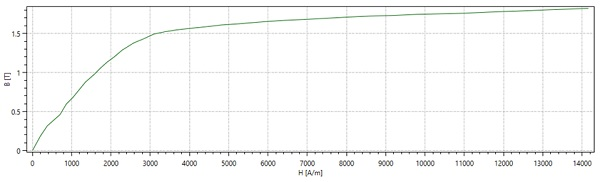Figure 8 - BH curve of Carbon steel .

Magnetic flux density, magnetic field intensity and magnetic potential results are generated by EMWorks2D. Figures9a) and 9b) contain respectively fringe and lines plot of the magnetic flux produced in the actuator. Equipotential contours of magnetic vector potential A is plotted in Figure 10.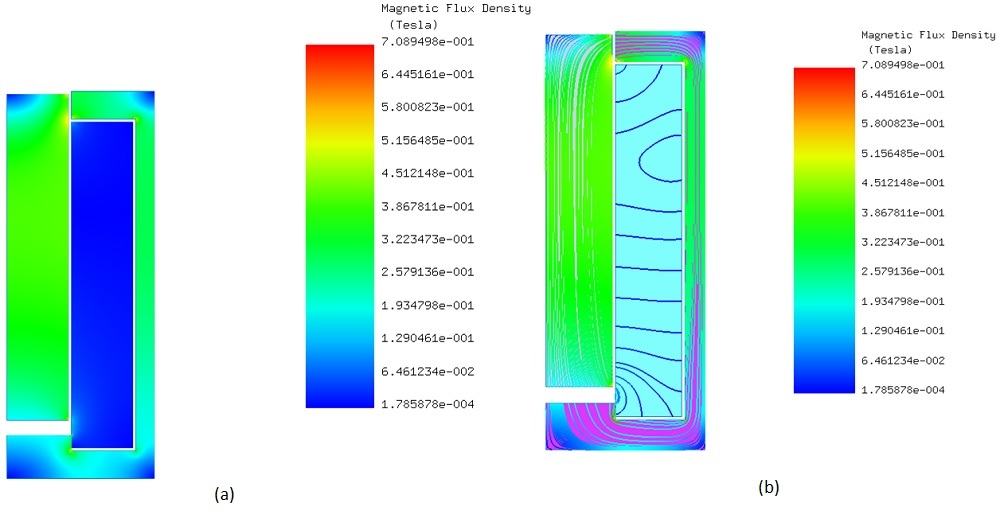Figure 9 - Magnetic flux density  a) fringe plot b) lines plot.

EMWorks2D is used to compute the force on the plunger versus both air gap distance and DC current. Using parameterization, the magnetic force curve is generated by varying the air gap distance. As mentioned above, the force inversely decreases with the air gap distance. Figure 11 contains a comparison of the computed force with EMWorks2D versus the reference ref.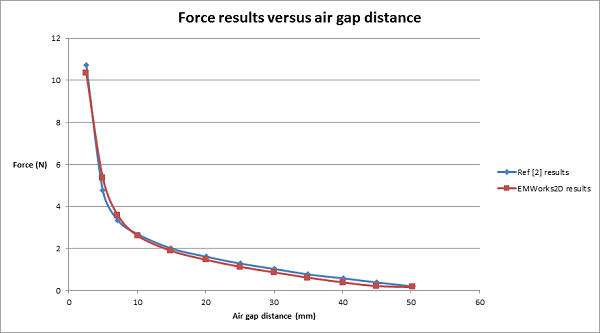Figure 11 -  Force results versus air gap distance.

By varying both current and air gap distance, EMWorks2D computes and generates the magnetic force in the actuator. Figure 12 shows 3D graph of the force results. The resultant force increases with higher current (N-I) and smaller gap distance.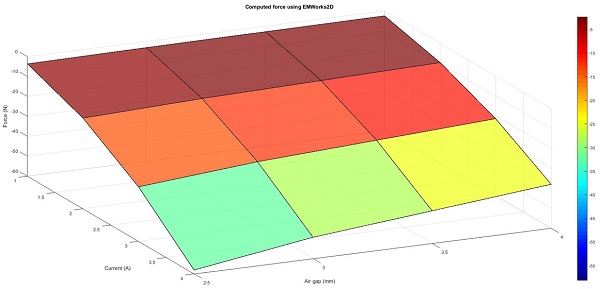Figure 12 - Force results versus air gap distance and applied current.

## Conclusion

The design and development of new generation of DC actuators is increasingly in demand because they are becoming widely used in several applications such as military and medical industry.

FEM simulation can be of a paramount help in achieving high accuracy and efficiency for such applications. To optimize and build efficient solenoid actuators, both 3D and 2D finite element analyses are used to compute magnetic forces, magnetic flux, current density, force density, etc. Although the 3D simulation is more accurate and takes into consideration more real-life conditions, 2D approximation is also used because it can help to save time and resources and accelerate the production process.

#### References

: https://www.electronics-tutorials.ws/io/io_6.html
: Se-Hee Lee, Hong-Soon Choi, and Il-Han Park .Introducing the Virtual Air-Gap Scheme to the Kelvin Force Densities With External and Total Field. IEEE TRANSACTIONS ON MAGNETICS, VOL. 43, NO. 4, APRIL 2007
: Terzova, A. I., V. M. Mateev, and I. Y. Marinova. Modelling of electromagnetic actuator with ferrofluid. CEMBEF 2013(2013): 75-78.